# Positive gaussoids

A positive gaussoid is an oriented gaussoid which assigns only the values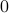and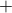. For each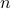there are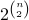positive gaussoids (Theorem 5.6).

## Enumeration

An oriented gaussoid is positive if all symbols have signor. Again, the cnf for positive gaussoids is a specialization to the positive case of the explicit listing of compatible assignments:

```((a=0)    ->(b=0&(c=0|d=0)))&
((a=+&b=0)->(c=+&d=+))
```

We use the canonical variable ordering and encode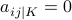if and only if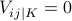.

 c2d time cachet time sharpSAT time bdd_minisat_all time pcnf3 8 0.00 * 0.01 * 0.00 pcnf3-list.txt 0.00 pcnf4 64 0.08 * 0.01 * 0.00 pcnf4-list.txt 0.00 pcnf5 1024 0.56 * 0.04 * 0.02 pcnf5-list.txt 0.06 pcnf6 32768 5.26 * 2.67 * 1.34 pcnf6-list.txt.bz2 5.05 pcnf7 2097152 1029 * 483 * 271 pcnf7-list.txt.bz2 750

The general formulafor the number of positive gaussoids was proved in Theorem 5.6.

To produce a listing of oriented gaussoids in the 0+- format, it suffices to replace 1 with + in the output of minisat2binary.pl:

```\$ minisat2binary.pl <pcnf3-list | tr '1' '+' >pcnf3-list.txt
```